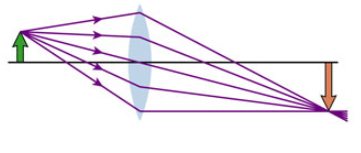# Problem: A converging lens creates the image shown in:Is the object distance less than the focal length f, between f and 2f, or greater than 2f?

🤓 Based on our data, we think this question is relevant for Professor Weliweriyan's class at UGA.

###### FREE Expert Solution

Magnification formula is:

$\overline{){\mathbf{m}}{\mathbf{=}}\frac{\mathbf{-}{\mathbf{s}}_{\mathbf{i}}}{{\mathbf{s}}_{\mathbf{o}}}}$

The image is real and inverted.

We have, from the figure, m = - 1.5

Therefore, si = - ms= -(-1.5)so = 1.5so###### Problem Details

A converging lens creates the image shown in:Is the object distance less than the focal length f, between f and 2f, or greater than 2f?